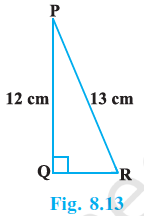Q

# In Fig. 8.13, find tan P - cot R.

2.     In Fig. 8.13, find .ViewsWe have, PQR is a right-angled triangle, length of PQ and PR are 12 cm and 13 cm respectively.
So, by using Pythagoras theorem,

Now, According to question,
=
= 5/12 - 5/12  = 0

Exams
Articles
Questions